# Moiré pattern

﻿
Moiré pattern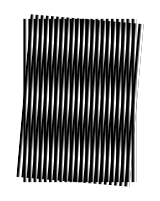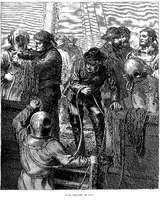A moiré pattern, formed by two sets of parallel lines, one set inclined at an angle of 5° to the other The fine lines that make up the sky in this image create moiré patterns in the sky when shown at some resolutions for the same reason that photographs of televisions exhibit Moire patterns: The lines are not absolutely level.

In physics, a moiré pattern (; French: [mwaʁe]) is an interference pattern created, for example, when two grids are overlaid at an angle, or when they have slightly different mesh sizes.

## Etymology

The term originates from moire (or moiré in its French form), a type of textile, traditionally of silk but now also of cotton or synthetic fiber, with a rippled or 'watered' appearance.

The history of the word moiré is complicated. The earliest agreed origin is the Arabic mukhayyar (مُخَيَّر in Arabic, which means chosen), a cloth made from the wool of the Angora goat, from khayyara (خيّر in Arabic), 'he chose' (hence 'a choice, or excellent, cloth'). It has also been suggested that the Arabic word was formed from the Latin marmoreus, meaning 'like marble'. By 1570 the word had found its way into English as mohair. This was then adopted into French as mouaire, and by 1660 (in the writings of Samuel Pepys) it had been adopted back into English as moire or moyre. Meanwhile the French mouaire had mutated into a verb, moirer, meaning 'to produce a watered textile by weaving or pressing', which by 1823 had spawned the adjective moiré. Moire (pronounced "mwar") and moiré (pronounced "mwar-ay") are now used somewhat interchangeably in English, though moire is more often used for the cloth and moiré for the pattern.

"Watered textile" refers to laying part of the textile on top of another part, and pressing the two layers when wet. The similarity of the spacing of individual threads (warp and woof), which is, however, not perfect spacing, creates characteristic patterns when the layers are pressed together; when dry, the patterns remain.

## Pattern formation

Moiré patterns are often an undesired artifact of images produced by various digital imaging and computer graphics techniques, for example when scanning a halftone picture or ray tracing a checkered plane (the latter being a special case of aliasing, due to undersampling a fine regular pattern). This can be overcome in texture mapping through the use of mipmapping and anisotropic filtering

The drawing on the upper right shows a moiré pattern. The lines could represent fibers in moiré silk, or lines drawn on paper or on a computer screen. The nonlinear interaction of the optical patterns of lines creates a real and visible pattern of roughly parallel dark and light bands, the moiré pattern, superimposed on the lines.

More complex line moiré patterns are created if the lines are curved or not exactly parallel. Moiré patterns revealing complex shapes, or sequences of symbols embedded in one of the layers (in form of periodically repeated compressed shapes) are created with shape moiré, otherwise called band moiré patterns. One of the most important properties of shape moiré is its ability to magnify tiny shapes along either one or both axes, that is, stretching. A common 2D example of moiré magnification occurs when viewing a chain-link fence through a second chain-link fence of identical design. The fine structure of the design is visible even at great distances.

## Calculations

### Moiré of parallel patterns

#### Geometrical approach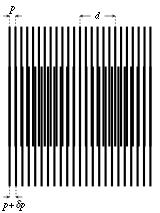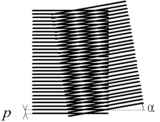the patterns are superimposed in the mid-width of the figure Moiré obtained by the superimposition of two similar patterns rotated by an angle α

Let us consider two patterns made of parallel and equidistant lines, e.g., vertical lines. The step of the first pattern is p, the step of the second is pp, with 0<δ<1.

If the lines of the patterns are superimposed at the left of the figure, the shift between the lines increase when going to the right. After a given number of lines, the patterns are opposed: the lines of the second pattern are between the lines of the first pattern. If we look from a far distance, we have the feeling of pale zones when the lines are superimposed, (there is white between the lines), and of dark zones when the lines are "opposed".

The middle of the first dark zone is when the shift is equal to p/2. The nth line of the second pattern is shifted by n·δp compared to the nth line of the first network. The middle of the first dark zone thus corresponds to

n·δp = p/2

that is$n = \frac{p}{2 \delta p}$.

The distance d between the middle of a pale zone and a dark zone is$d = n \cdot p = \frac{p^2}{2 \delta p}$

the distance between the middle of two dark zones, which is also the distance between two pale zones, is$2d = \frac{p^2}{\delta p}$

From this formula, we can see that :

• the bigger the step, the bigger the distance between the pale and dark zones;
• the bigger the discrepancy δp, the closer the dark and pale zones; a great spacing between dark and pale zones mean that the patterns have very close steps.

Of course, when δp = p/2, we have a uniformly grey figure, with no contrast.

The principle of the moiré is similar to the Vernier scale.

#### Interferometric approach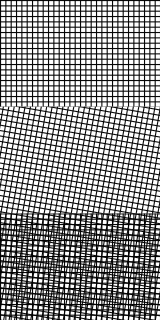Moiré pattern (bottom) created by superimposing two grids (top and middle). See full-size image to see moiré pattern more distinctly.

Let us consider now two transparent patterns with a contrast I that varies with a sinusoidal law:$I_1(x) = I_0 \cdot \sin (2\pi \cdot k_1 \cdot x)$$I_2(x) = I_0 \cdot \sin (2\pi \cdot k_2 \cdot x)$

(the steps are respectively p1 = 1/k1 and p2 = 1/k2), when the patterns are superimposed, the resulting intensity (interference) is$I(x) = I_0 \cdot ( \sin (2\pi \cdot k_1 \cdot x) + \sin (2\pi \cdot k_2 \cdot x) )$

with the Euler's formula:$I(x) = I_0 \cdot 2 \cos \left ( 2\pi \frac{(k_1-k_2)}{2} \cdot x \right ) \cdot \sin \left ( 2\pi \frac{(k_1+k_2)}{2} \cdot x \right )$

We can see that the resulting intensity is made of a sinus law with a high "spatial frequency" (wave number) which is the average of the spatial frequencies of the two patterns, and of a sinus law with a low spatial frequency which is the half of the difference between the spatial frequencies of the two patterns. This second component is an "envelope" for the first sinus law. The wavelength λ of this component is the inverse of the spatial frequency$\frac{1}{\lambda} = \frac{k_1 - k_2}{2} = \frac{1}{2} \cdot \left ( \frac{1}{p_1} - \frac{1}{p_2} \right )$

if we consider that's p1 = p and p2 = pp:$\lambda = 2\frac{p_1 p_2}{p_2 - p_1} \approx 2\frac{p^2}{\delta p}$.

The distance between the zeros of this envelope is λ/2, and the maxima of amplitude are also spaced by λ/2; we thus obtain the same results as the geometrical approach, with a discrepancy of p/2 which is the uncertainty linked to the reference that is considered: pattern 1 or pattern 2. This discrepancy is negligible when δp << p.

This phenomenon is similar to the stroboscopy.

### Rotated patterns

Let us consider two patterns with the same step p, but the second pattern is turned by an angle α. Seen from far, we can also see dark and pale lines: the pale lines correspond to the lines of nodes, that is, lines passing through the intersections of the two patterns.

If we consider a cell of the "net", we can see that the cell is a rhombus: it is a parallelogram with the four sides equal to d = p/sin α; (we have a right triangle which hypothenuse is d and the side opposed to the α angle is p).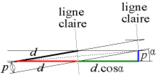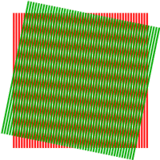Unit cell of the "net"; "ligne claire" means "pale line" Effect of changing angle.

The pale lines correspond to the small diagonal of the rhombus. As the diagonals are the bisectors of the neighbouring sides, we can see that the pale line makes an angle equal to α/2 with the perpendicular of the lines of each pattern.

Additionally, the spacing between two pale lines is D, the half of the big diagonal. The big diagonal 2D is the hypothenuse of a right triangle and the sides of the right angle are d(1+cos α) and p. The Pythagorean theorem gives:

(2D)2 : d2(1+cos α)2 + p2

id est$(2D)^2 = \frac{p^2}{\sin^2 \alpha}(1+ \cos \alpha)^2 + p^2 = p^2 \cdot \left ( \frac{(1 + \cos \alpha)^2}{\sin^2 \alpha} + 1\right )$

thus$(2D)^2 = 2 p^2 \cdot \frac{1+\cos \alpha}{\sin^2 \alpha}$

When α is very small (α < π/6), the following approximations can be done:

sin α ≈ α
cos α ≈ 1

thus

Dp / α.

We can see that the smaller the α, the farthest the pale lines; when the both patterns are parallel (α = 0), the spacing between the pale lines is "infinite" (there is no pale line).

There are thus two ways to determine α: by the orientation of the pale lines and by their spacing

α ≈ p / D

If we choose to measure the angle, the final error is proportional to the measurement error. If we choose to measure the spacing, the final error is proportional to the inverse of the spacing. Thus, for the small angles, it is best to measure the spacing.

## Implications & applications

### Printing full-color imagesThe product of two "beat tracks" of slightly different speeds overlaid, producing an audible moiré pattern; if the beats of one track correspond to where in space a black dot or line exists and the beats of the other track correspond to the points in space where a camera is sampling light, because the frequencies are not exactly the same and aligned perfectly together, beats (or samples) will align closely at some moments in time and far apart at other times. The closer together beats are, the darker it is at that spot; the farther apart, the lighter. The result is periodic in the same way as a graphic moiré pattern.

In graphic arts and prepress, the usual technology for printing full-color images involves the superimposition of halftone screens. These are regular rectangular dot patterns—often four of them, printed in cyan, yellow, magenta, and black. Some kind of moiré pattern is inevitable, but in favorable circumstances the pattern is "tight;" that is, the spatial frequency of the moiré is so high that it is not noticeable. In the graphic arts, the term moiré means an excessively visible moiré pattern. Part of the prepress art consists of selecting screen angles and halftone frequencies which minimize moiré. The visibility of moiré is not entirely predictable. The same set of screens may produce good results with some images, but visible moiré with others.

In manufacturing industries, these patterns are used for studying microscopic strain in materials: by deforming a grid with respect to a reference grid and measuring the moiré pattern, the stress levels and patterns can be deduced. This technique is attractive because the scale of the moiré pattern is much larger than the deflection that causes it, making measurement easier.

### Television screens and photographs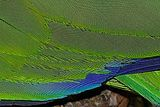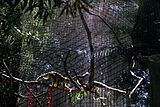Strong moiré visible in this photo of a parrot's feathers (more pronounced in the full-size image) Moiré Fringes (Pattern) are seen over a cage in the San Francisco Zoo

Moiré patterns are commonly seen on television screens directly when a person is wearing a shirt or jacket of a particular weave or pattern, such as a houndstooth jacket, due to sampling problems in the television camera. As the person moves about, the Moiré pattern is quite noticeable. Because of this, newscasters and other professionals who appear on TV regularly are instructed to avoid clothing which could cause the effect, more commonly known as 'strobing'.

Photographs of a TV screen taken with a digital camera often exhibit moiré patterns.

The Moiré effect is used in shoreside beacons to mark underwater hazards (usually pipelines or cables). The Moiré effect creates arrows that 'point' towards an imaginary line marking the hazard. As you pass over the hazard, the arrows on the beacon appear to become vertical bands before 'changing' back to arrows pointing in the reverse direction. An example can be found in the UK on the East shore of Southampton water, opposite Fawley oil refinery (50°51′21.63″N 1°19′44.77″W﻿ / ﻿50.8560083°N 1.3291028°W). Similar Moiré effect beacons can be used to guide mariners to the centre point of an oncoming bridge (here you would position the vessel so that you could see vertical lines - denoting the 'centreline').

### Strain measurementUse of the moiré effect in strain measurement: case of uniaxial traction (top) and of pure shear (bottom); the lines of the patterns are initially horizontal in both cases

The moiré effect can be used in strain measurement: the operator just has to draw a pattern on the object, and superimpose the reference pattern to the deformed pattern on the deformed object.

A similar effect can be obtained by the superposition of an holographic image of the object to the object itself: the hologram is the reference step, and the difference with the object are the deformations, which appear as pale and dark lines.

### Image processing

Some image scanner driver programs provide an optional filter, called a "descreen" filter, to remove Moiré-pattern artifacts which would otherwise be produced when scanning printed halftone images to produce digital images.

Wikimedia Foundation. 2010.

### Look at other dictionaries:

• moiré pattern — moiré effect or moiré pattern noun An optical effect, a shifting wavy pattern seen when two surfaces covered with regular lines are superimposed • • • Main Entry: ↑moire …   Useful english dictionary

• moiré pattern — ▪ physics  in physics, the geometrical design that results when a set of straight or curved lines is superposed onto another set; the name derives from a French word for “watered.” The effect may be seen by looking through the folds of a nylon… …   Universalium

• moire pattern — muaro piešinys statusas T sritis informatika apibrėžtis Vaizdas, primenantis banguotą raibuliuojantį paviršių, susidarantis dėl šviesos interferencijos, kai vienas ant kito ne visai tiksliai projektuojami tapatūs piešiniai. Iliustraciją žr.… …   Enciklopedinis kompiuterijos žodynas

• moiré pattern — noun an optical effect formed when one family of curves is superposed on another, the lines of the overlapping figures crossing at an ange less than about 45°; the moiré lines are then the locus of the points of intersection …   Wiktionary

• Moire (fabric) — Moire ribbons In textiles, a moire (pronounced /ˈmwɑr/ or /ˈmɔr/) (less often, moiré) is a fabric with a wavy (watered) appearance produced mainly from silk, but also wool, cotton and rayon. The watered ap …   Wikipedia

• Moire (disambiguation) — Moire or Moiré may refer to: Emmanuel Moire (born 1979), singer Line moiré Moire (fabric) Moire deflectometry Moiré pattern Moiré, Rhône Shape moiré See also Moir (disambiguation) Moires, Greece …   Wikipedia

• moiré effect — n. Optics. the appearance, when two regularly spaced sets of lines are superimposed, of a new set of lines (moiré pattern) passing through the points where the original lines cross at small angles. [1950 55] * * * moiré effect or moiré pattern… …   Useful english dictionary

• moiré effect — n. Optics. the appearance, when two regularly spaced sets of lines are superimposed, of a new set of lines (moiré pattern) passing through the points where the original lines cross at small angles. [1950 55] * * * …   Universalium

• moiré — [mwä rā′, môrā′; môr′ā] adj. [Fr, pp. of moirer, to water < moire: see MOIRE] having a watered, or wavy, pattern, as certain fabrics, stamps, or metal surfaces n. 1. a watered pattern pressed into cloth, etc. with engraved rollers 2. MOIRE …   English World dictionary

• Moire deflectometry — Moiré Deflectometry is an interferometry technique, in which the object to be tested (either phase object or secular surface) is mounted in the course of a collimated beam followed by a pair of transmission gratings placed at a distance from… …   Wikipedia